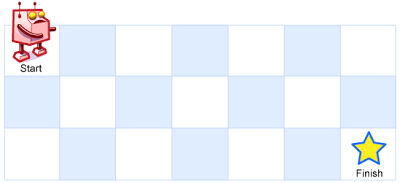# 什么是动态规划——从青蛙跳台阶开始了解

nill0705

0收藏

Hello 大家好，我是阿粉，动态规划（Dynamic Programming），简称 DP 相信大家在日常的工作或者学习的过程中都遇到过这个词，而且动态规划也是面试过程中最喜欢被问到的题目，阿粉在经历的不多的几场面试中都被问到了，实在是苦不堪言，不过好在阿粉还是有学过的，一些简单的套路阿粉还是懂的。下面就从一个很多人应该都不陌生的题目讲起。

### 编码

``````public int dp(int n) {
if (n <= 0) {
return 0;
}
int[] dp = new int[n + 1];
dp = 0;
dp = 1;
dp = 2;
// 之所以要从 3 开始，是因为 2 不符合下面的规则
for (int i = 3; i <= n; i++) {
dp[i] = dp[i - 1] + dp[i - 2];
}
return dp[n];
}``````

### 分析

1. 定义变量：把已知的和需要求解的，定义出变量，如上面的​`​n​`​​ 和​`​f(n)​`​；
2. 寻找表达式：找到​`​f(n)​`​​ 和​`​f(n - 1)​`​​ 以及​`​f(n - 2)​`​​，等情况的表达式，如上面的​`​f(n) = f(n - 1) + f(n - 2)​`​，这一步往往是最难的；
3. 寻找初始值：确保找到所有的临界条件，如上面的​`​f(0) = 0, f(1) = 1, f(2) = 2​`​；

### 案例 2img

1. 定义​`​d[m][n]​`​​ 表示在​`​m x n​`​ 网格上移到右下角需要的总步数；
2. 因为机器人只能向右和向下移动，所以到达下一个格子只能是从左边或者上面，所以达到​`​m x n​`​​ 的步数等于​`​(m - 1) x n + m x (n - 1)​`​​，也就是​`​d[m][n] = d[m - 1][n] + d[m][n - 1]​`​；
3. 定义初始值​`​d[n] = 1​`​​，​`​d[n] = 1​`​，也就是只有一行或者一列的时候只有一种方法，全部向下或者向右移动；

### 编码

``````public int dp(int m, int n) {
if (m <=0 || n <= 0) {
return 0;
}
int[][] dp = new int[m][n];
//只有一列的时候
for (int i = 0; i < m; i++) {
d[i] = 1;
}
//只有一行的时候
for (int i = 0; i < n; i++) {
d[i] = 1;
}

for (int i = 1; i < m; i++) {
for (int j = 1; j < n; j++) {
d[i][j] = d[i][j - 1] + d[i - 1][j];
}
}
//数组的下标从 0 开始
return d[m - 1][n - 1];
}``````

### 总结帖子
视频
声望
粉丝
社区精华内容